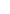## Amplifiers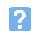1. An amplifier covers the frequency range 1MHz to 3MHz. Which of the following most correctly describes this type of amplifer?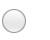a) A wideband amplifierb) An a.c. coupled amplifierc) A radio frequency amplifierd) An audio frequency amplifier.2. An amplifier provides an output of 2.5V for an input of 12.5mV. Which one of the following gives the voltage gain of the amplifier?a) 20b) 50c) 80d) 200.3. The bias point for an amplifier is set at projected cut-off. The corresponding class of operation is::a) Class Ab) Class ABc) Class Bd) Class C.4. The class of operation most appropriate for a high-efficiency RF amplifier is:a) Class Ab) Class ABc) Class Bd) Class C.5. An amplifier has an open-loop gain of 10 and 10% of the output is fed back to the input as negative feedback. Which one of the following gives the gain with negative feedback applied?a) 1b) 5c) 9d) 10.6. Which operating configuration is used in the diagram shown below?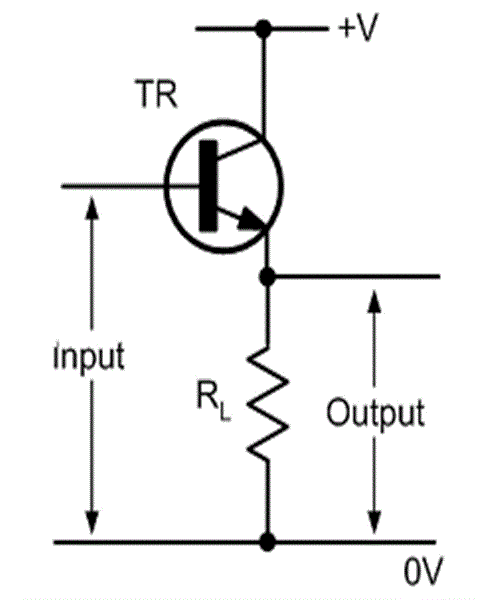a) Common baseb) Common sourcec) Common emitterd) Common collector.7. In the circuit shown below, the function of R1 is: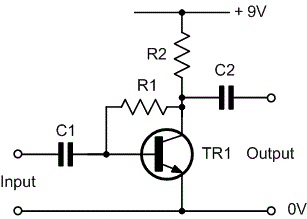a) to supply base bias currentb) to provide additional smoothingc) to increase the overall voltage gain of the amplifierd) to act as a load across which the output voltage is developed.8. In the diagram shown below, which one of the following gives the optimum value for the collector voltage for TR1?",2,a) 0.6Vb) 2.2Vc) 4.5Vd) 8.4V.9. Which one of the following gives the correct adjustment for RV1 in the diagram below?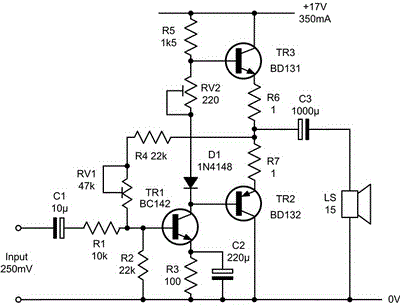a) Setting the voltage gain to exactly 50b) Setting the quiescent supply current to about 300 mAc) Setting the voltage at the junction of R6 and R7 to 8.5Vd) Setting the signal volume to a suitable level for the loudspeaker.10. In the circuit shown below, what will happen if C2 is open-circuit?a) The supply current will increaseb) The amplifier will become unstablec) The output will become very distortedd) The output volume will be slightly reduced.
This is more feedback!
This is the feedback!